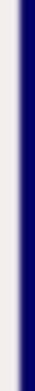homework help forum today's math problem links utilities previous math problem feedback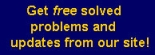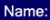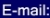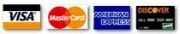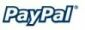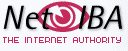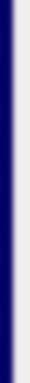# MATH HOMEWORK HELP

8. After x weeks the number of persons using a new drug was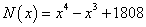. At what percentage rate was the use of the drug changing after four weeks?

Solution: Let’s compute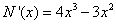We can take two approaches: by derivatives (approximation) or exactly:

• Derivatives:

After four weeks the derivative is computed as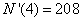which means that approximately 208 new people a week use the drug after four weeks. We also know that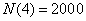the percentage rate the use of the drug is changing after four weeks is 10.4% (approximation using derivatives).

• Exactly:

We can compute the percentage exactly as: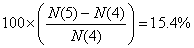(b) By how much did the number of persons using this drug actually change during the fourth week?

Solution: We can find the exact change computing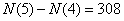MGT - Your source of Math and Statistics Homework Help